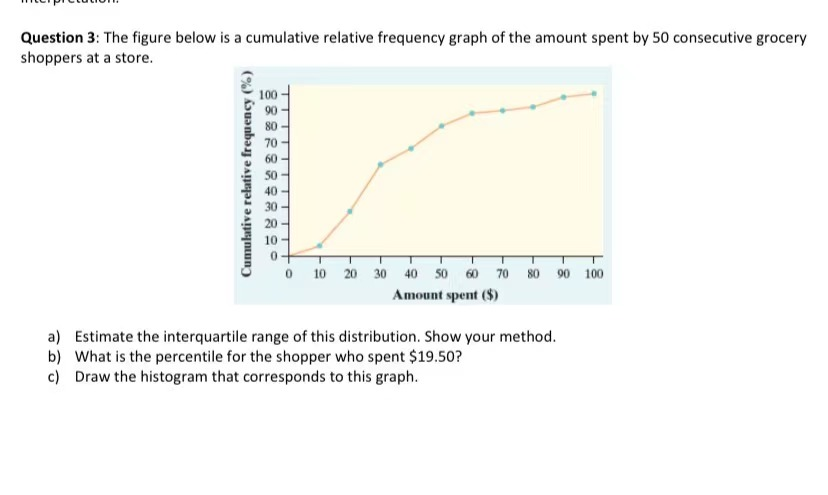💬 👋 We’re always here. Join our Discord to connect with other students 24/7, any time, night or day.Join Here!

MW

Michelle W.

Intro Stats / AP Statistics

1 week, 5 days ago

# The figure below is a cumulative relative frequency graph of the amount spent by 50 consecutive grocery shoppers at a store.Other Schools

The figure below is a cumulative relative frequency graph of the amount spent by 50 consecutive grocery shoppers at a store.

### Discussion

You must be signed in to discuss.

### Video Transcript

Hello Suit. I'm going to answer your question about application of cumulative distribution function in a rosary topic. For doing so. First I used this rough and then I start answering questions. My question. So the first question will be to calculate intercultural range. That is the difference between the third quarter. Also the that 75% and the first quarter till but he said 25%. So this will be for 25%. It will be for 75%. How I estimate it is that I go to the vertical axis and The one for the honorees underlining what I am that is 25% and I row there isn't until it crosses with this graph. Then I go down to see what value it is. So at 25% value. I would estimate this 19 is where this 19 come from. They may do the same process for 75. For the third quarter two. I draw on a horizontal line until it crossed with the graph. And then I put it To see what is the amount of money in that specific presented in this case. I am to be 49. Then I make a difference. And this history. The next question is to calculate the percentage of 19.50. In this case I use the red lines that you see here. So the way that I do it is is that I situate myself in $19.50. Then I grew a vertical line until I crossed with this graph. And when I crossed with this rough I draw a vertical and horizontal line to see which percentage it is. And I will estimate that is Between that said 26 or 27 and 28%. So this will be these values and the result will be between these values because it's hard to say and a specific value without the scale. Then they tell you that you need to build an instagram. So the way you build it, I just give you a rough example here. Maybe if I make it a little bit smaller so what you need to is to start checking how much information is in each mean. So what I did is the percentages here and the money is in the horizontal in the horizontal axis. So I will fill five beans from 0 to$20.20 to $40 and so on. So what we said is that between$0-$20 is almost 30, right? Almost 30% of that. So that's where this story come from them from$20 To \$40. So you will need to subtract the value from 40 that I estimate. That is around charity. So I will say that 30 minutes for him. three million 70. Sorry, it's 40. So that's where it is for you come from. And then I keep doing this in process of now from 40 to 60. So I have that it's around 70 and 60s. Around Let's say 80 or 90. So you would put the value between 19 or 18 and 20 and so on and that's the way you build an instagram. So this is a consumer. Remember that they build to this diagram is subtracting the previous cumulative values to the next value. So you can have the cumulative distribution. But just the distribution and this gives you a better understanding of how much it's in ich bin ich bin is the range of money that you can establish Arbitrarily. Also that the way that you estimate the percentage using the cumulative distribution is starting from the horizontal axis, then growing a vertical line and then we'll see where it cruises. You can use the red lines as a reference And finally intercounty ranges. Everything between the third quarter or the percent of 75 and the first quarter or the percent of 25. I do it their way around. You can use the black lines to use a reference. So for example in the case for 75 I threw a vertical line where I estimate that 75%. Then I checked where it closes, it closes in this point and then I draw a vertical line to see what value of money is. According to me. That 49. I hope definition was clear and think pretty much for your timeOther Schools
##### Top Intro Stats / AP Statistics Educators##### Catherine R.

Missouri State University##### Michael J.

Idaho State UniversityLectures

Join Bootcamp